### Representation Learning on Graphs with Jumping Knowledge Networks

Keyulu Xu1, Chengtao Li1, Yonglong Tian1, Tomohiro Sonobe2, Ken-ichi Kawarabayashi2, Stefanie Jegelka1

1. Massachusetts Institute of Technology (MIT)

2. National Institute for Informatics, Tokyo;

2018

presented by Albert M Orozco Camacho

# Motivation

Neighborhood aggregation turns out to be a crucial part of representation learning, due to the rise of graph neural networks.

Such procedure aims to extract high-level features from nodes via a message passing scheme.

### Caveats of Traditional Aggregation Schemes

Networks that exhibit a diversity in subgraph structures (such as node hubs) yield inconsistent learning of node relations by GCNs.

## Neighborhood aggregation in GNNs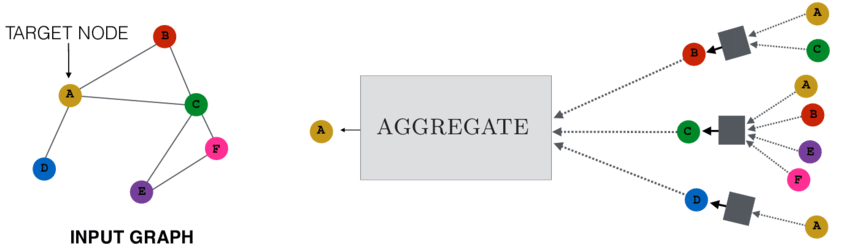## Influence distributions vs Random Walks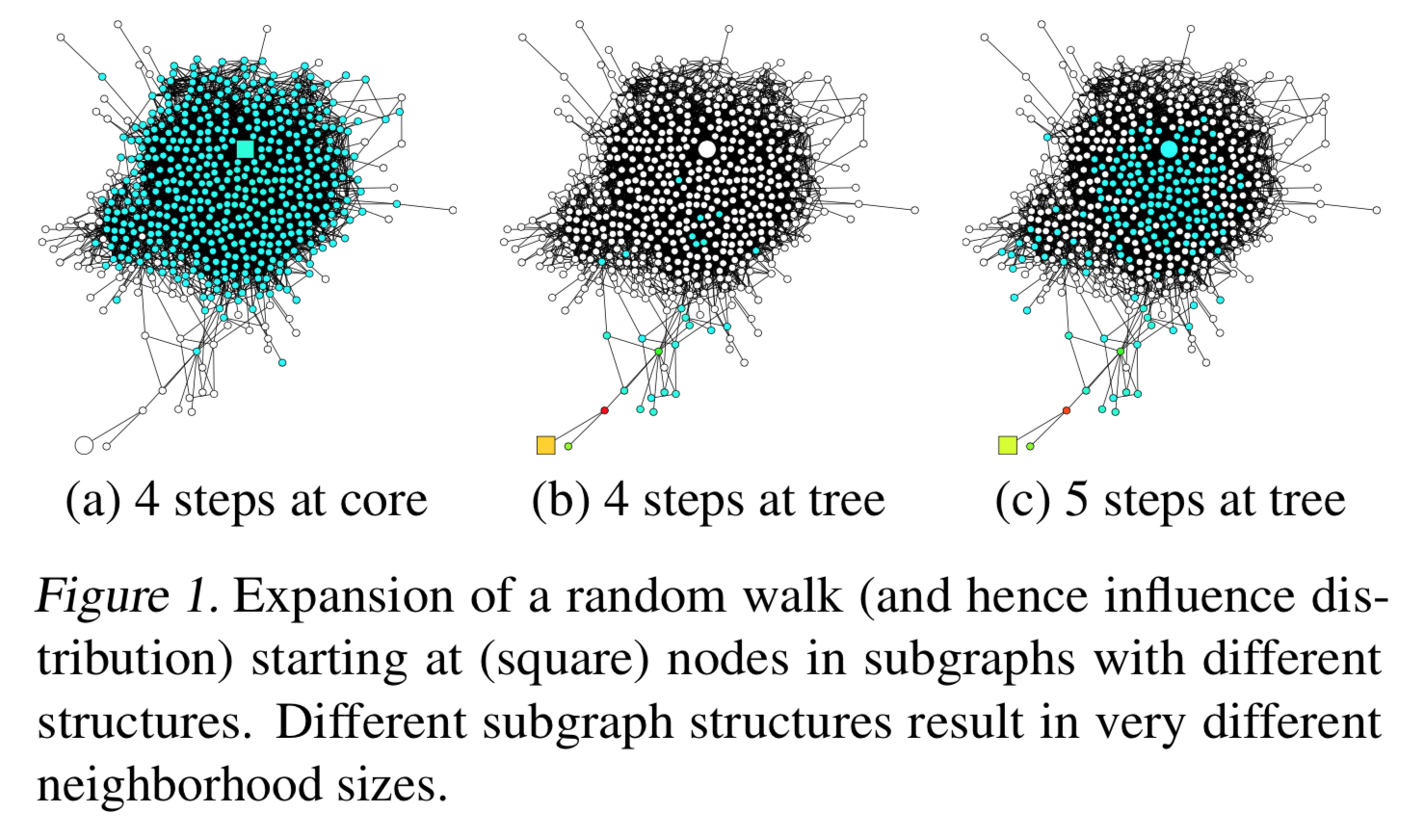# Background

### Hidden Layer Update for a GNN

$$h_v^{(l)} = \sigma\left(W_l \cdot \text{AGGREGATE}\left(\{h_u^{(l-1)}, \forall u \in \tilde{N}(v)\}\right)\right)$$

### Graph Convolutional Neural Networks

$$h_v^{(l)} = \text{RELU}\left(W_l \cdot \sum_{u \in \tilde{N}(v)}(\deg(v)\deg(u))^{-\frac{1}{2}} h_u^{(l-1)}\right)$$

$$h_v^{(l)} = \text{RELU}\left(W_l \cdot \frac{1}{\tilde{\deg}(v)} \sum_{u \in \tilde{N}(v)} h_u^{(l-1)}\right)$$

### Neighborhood Aggregation with Skip Connections

$$h_{N(v)}^{(l)} = \sigma\left(W_l \cdot \text{AGGREGATE}_N\left(\{h_u^{(l-1)}, \forall u \in N(v)\}\right)\right)$$

$$h_v^{(l)} = \text{COMBINE}\left(h_v^{(l-1)}, h_{N(v)}^{(l)}\right)$$

## Influence Distributions

$$I_x(y) = \frac{e^T \left[\frac{\partial h_x^{(k)}}{\partial h_y^{(0)}} e\right]}{\sum_{z \in V} e^T \frac{\partial h_x^{(k)}}{\partial h_z^{(0)}} e}$$

where $e$ is an all-ones vector

# Jumping Knowledge Networks

### The proposed aggregation scheme...

makes each layer increase the size of the influence distribution by aggregating neighborhoods from the previous layer ⬆️;

combines, at the last layer, some of the previous layers' representations independently for each node;

### Aggregation Mechanisms

CONCATENATION $$[h_v^{(1)},\ldots,h_v^{(k)}]$$

MAX-POOLING. Select the most informative layer for each feature coordinate.

LSTM-ATTENTION. Input $h_v^{(1)},\ldots,h_v^{(k)}$ into a bi-directional LSTM to generate forward and backward features $f_v^{(l)}$ and $b_v^{(l)}$ for each layer $l$; finally compute an attention score per each node by combining those for each layer.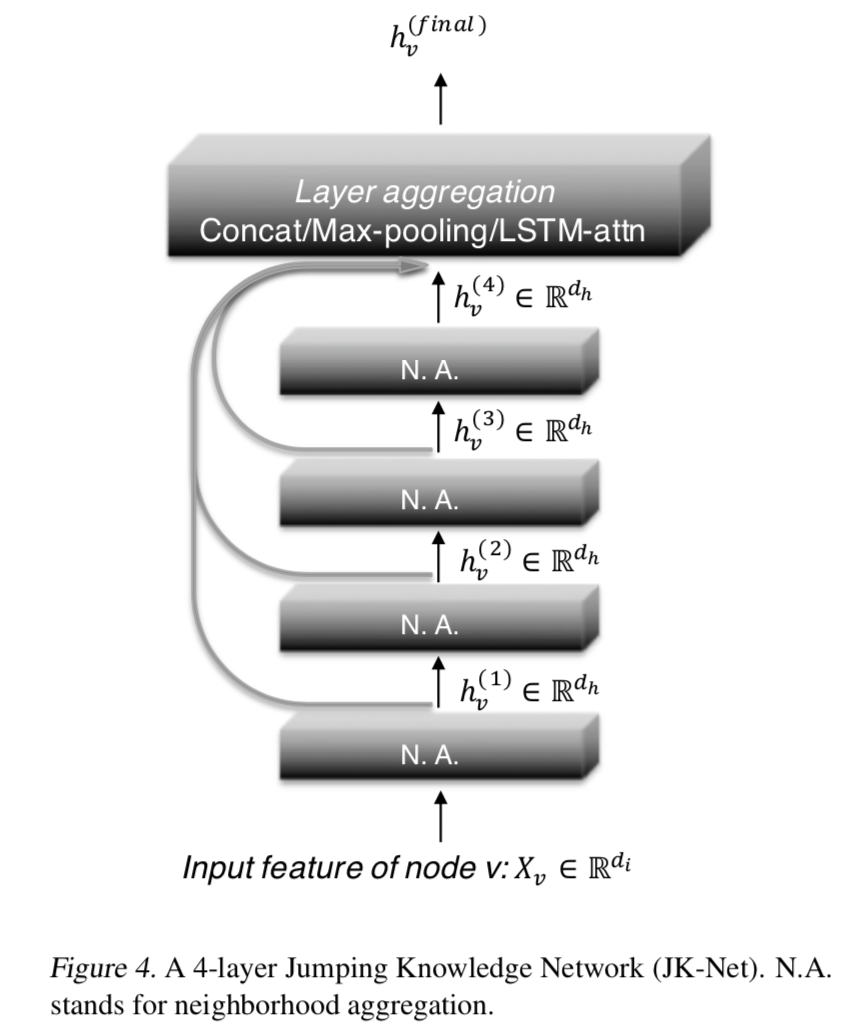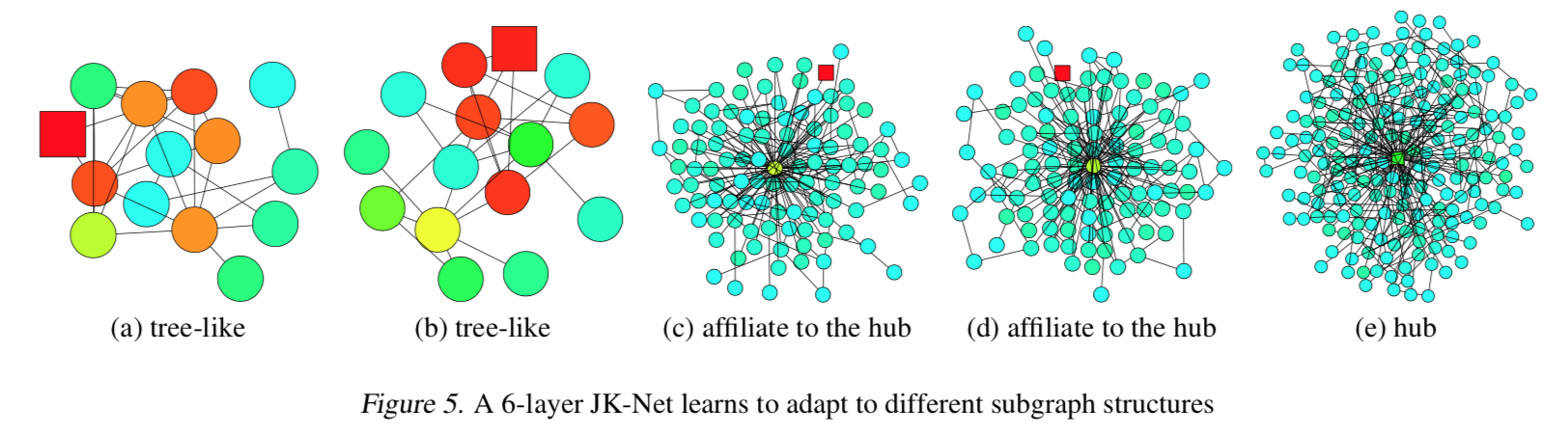### Proposition 1

Assume that paths of the same length in the computation graph are activated with the same probability.

The influence score $I(x, y)$ for any $x, y \in V$ under a $k$-layer JK-Net with layer-wise max-pooling is equivalent in expectation to a mixture of $0,\ldots,k$-step random walk distributions on $\tilde{G}$ at $y$ starting at $x$, the coefficients of which depend on the values of the layer features $h_x^{(l)}$.

# Experiments

### Datasets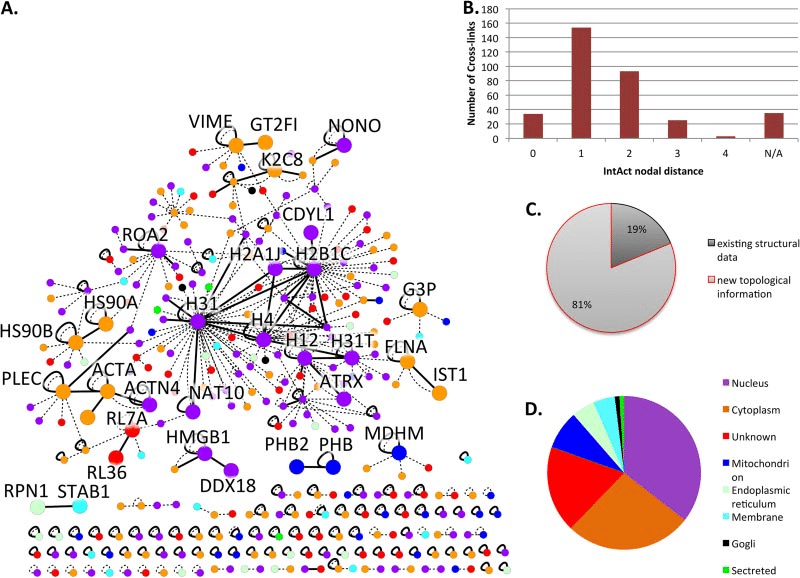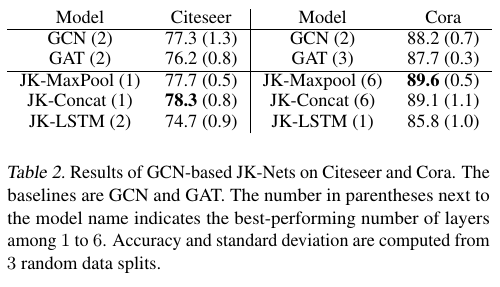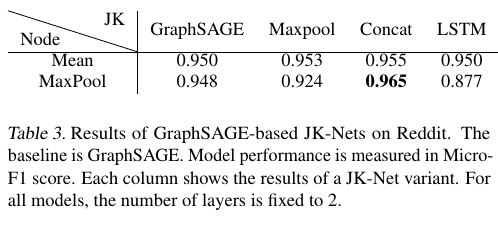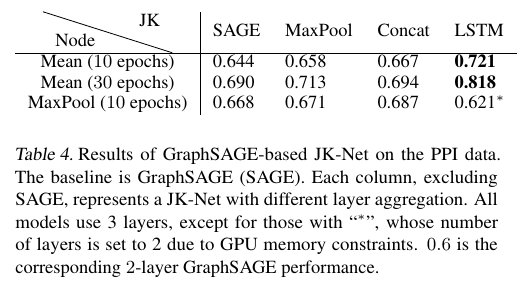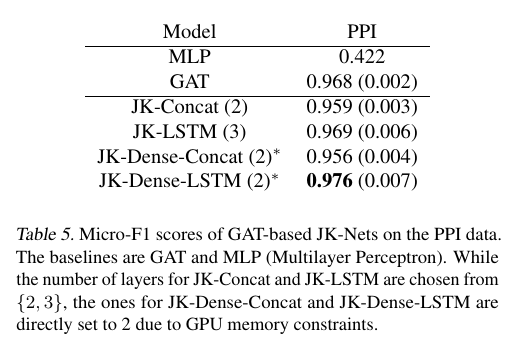# Conclusion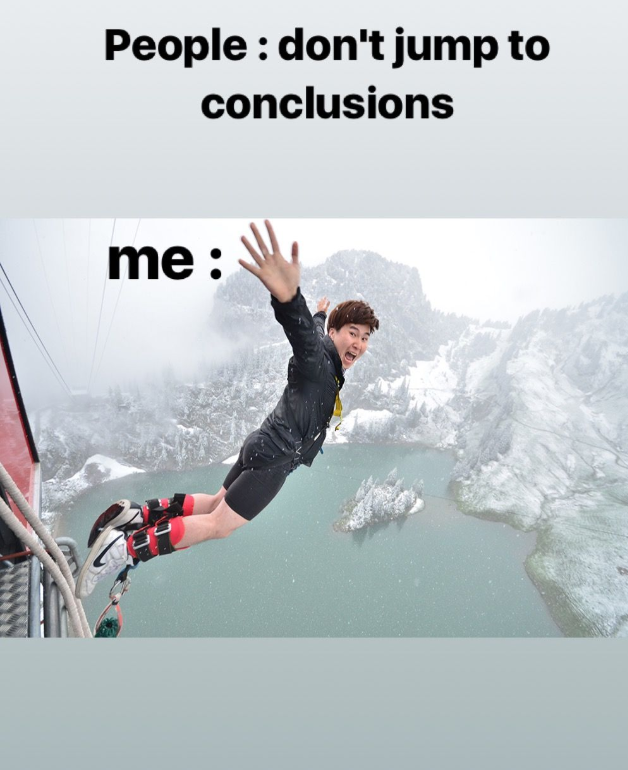Goal: Provide a representation learning scheme that can generalize better on diverse variety of network structure, than the one proposed for GCN's

Problem: Denser subgraphs may cause aggregation algorithms to converge in expectation to biased random walks. ☹

Solution: JK-Nets aggregate and leverage information from more than one hidden layers.😁

JK-Nets with the LSTM-attention aggregators outperform the non-adaptive models GraphSAGE, GAT and JK-Nets with concatenation aggregators.

### Future Work

Exploring other layer aggregators and studying the effect of the combination of various layer-wise and node-wise aggregators on different types of graph structures.

How can sequence modelling by itself impact the task of layer aggregation?

Are there smarter ways to keep track of node/community correlations within a network?